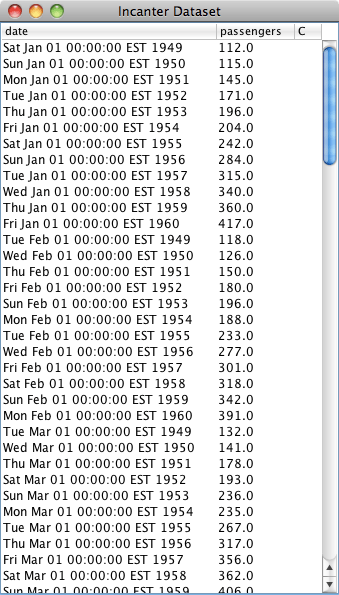# Category Archives: xls

## Reading and writing Excel (xls) files with Incanter

I have just added David James Humphreys’ incanter-excel module to the Incanter distribution, providing basic capabilities for reading Microsoft Excel spreadsheets in as Incanter datasets and saving datasets back out as xls files.

I have posted a simple spreadsheet of Australian airline passenger data from the 1950s to the Incanter website for the following example. The read-xls function can read xls files given either a filename or URL, so you won’t need to download the file.```(use '(incanter core charts excel))
```

Next, we can use the with-data macro to bind a dataset converted from the above xls file, using the read-xls function, and then view it.

```(with-data (read-xls "http://incanter.org/data/aus-airline-passengers.xls")
(view \$data)
```The read-xls function takes an optional argument called :sheet that takes either the name or index of the worksheet from the xls file to read (in this case either “dataset” or 0) , it defaults to 0.

[NOTE: A current weakness of read-xls is that cells containing formulae, as opposed to actual data, are not imported (i.e. the cells remain empty).]

Finally, we’ll create a time-series plot of the data. However, the time-series-plot needs time in milliseconds, so we’ll first create a function that converts the date column from Java Date objects to milliseconds, and then view the plot.

```  (let [to-millis (fn [dates] (map #(.getTime %) dates))]
(view (time-series-plot (to-millis (\$ :date)) (\$ :passengers)))))
```Datasets can also be saved as Excel files using the save-xls function. The following example just reads in one of the sample datasets using incanter.datasets/get-dataset and then saves it as an xls file.

```(save-xls (get-dataset :cars) "/tmp/cars.xls")
```

The incanter-excel module is now included in the Incanter distribution on Github, and is available as a separate dependency from the Clojars repository. The complete code from this example can be found here.